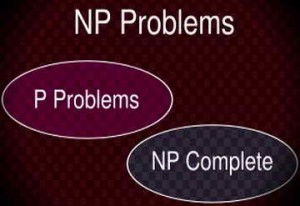# Epistemic Agents In The Context Of The P=?NP ProblemP=?NP is a famous problem common for mathematical logic and mathematical theory of computational complexity. Computational complexity theory divides formal problems in different classes according to the function that describes the output of the computational machine for the given formula as an input. There are several classes that are combined into a complex hierarchy which though has blank spaces. Continue reading